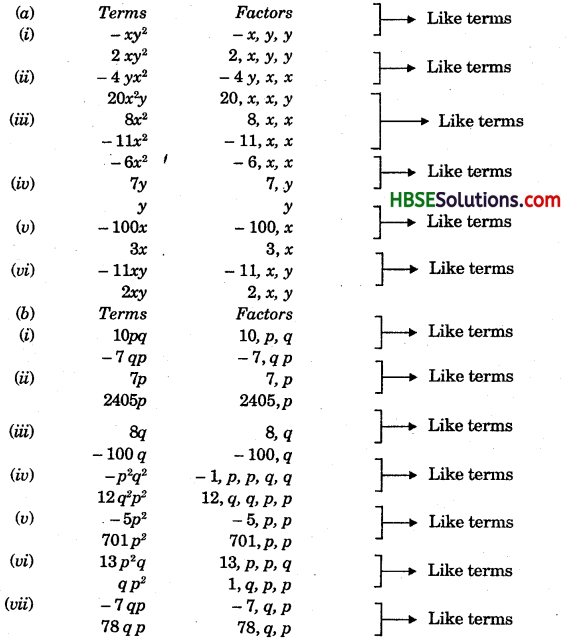# HBSE 7th Class Maths Solutions Chapter 12 Algebraic Expressions Ex 12.1

Haryana State Board HBSE 7th Class Maths Solutions Chapter 12 Algebraic Expressions Ex 12.1 Textbook Exercise Questions and Answers.

## Haryana Board 7th Class Maths Solutions Chapter 12 Algebraic Expressions Exercise 12.1

Question 1.
Get the algebraic expressions in the following cases using variables, constants and arithmetic operations.
(i) Subtraction of z from y.
(ii) One half of the sum of number x and y.
(iii) The number z multiplied by itself.
(iv) One fourth of the product of number p and q.
(v) Number x and y both squared and added.
(vi) Number 5 added to three times and product of number m and n.
(vii) Product of numbers y and z subtracted from 10.
(viii) Sum of numbers a and b subtracted from their product.
Solution:
(i) y -z
(ii) $$\frac{1}{2}$$ (x + y)
(iii) z x z =z2
(iv) $$\frac{1}{4}$$ (p x q) = $$\frac{1}{4}$$ (pq) = $$\frac{pq}{4}$$
(v) x2 + y2
(vi) 3mn + 5
(vii) 10 – yz
(viii) ab – (a + b)Question 2.
(i) Identify the terms and their factors in the foUowing expressions. Show the terms and factors by tree diagrams.
(a) x – 3
(b) 1 + x + x2
(c) y – y3
(d) 5xy2 + 7x2y
(e) -ab + 2b2 – 3a2
(ii) Identify terms and factors in the expressions given below:
(a) -4x + 5
(b) -4x + 5y
(e) 5y + 3y2
(d) xy + 2x2y2
(e) pq+q
(f) 1.2ab – 2.4b + 3.6a
(g.) $$\frac{3}{4} x+\frac{1}{4} h$$
(h) $$\frac{l+m}{5}$$
[Hint: Separate l and m terms]
(j) 0.1p2 + 0.2q2
(j) $$\frac{3}{4}$$ (a – b) + $$\frac{7}{4}$$
[Hint: Open the brackets]
Solution:
(i) (a) x – 3: In this expression x – 3 consists two terms x and -3.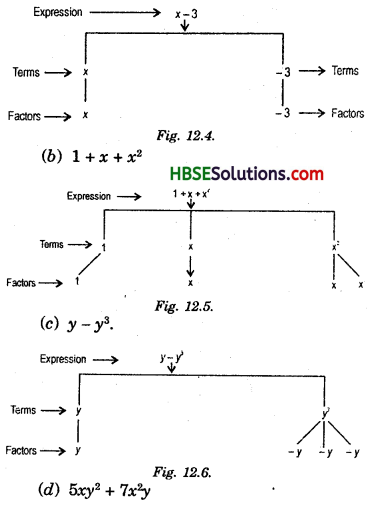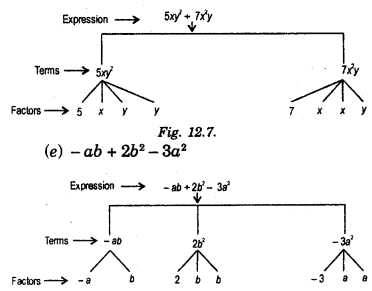(ii) (a) – 4x + 5.
The expression (- 4x + 5) consists of two terms – 4x and 5. The term – 4x is product of – 4 and x. And the term 5 has only one factor that is 5.
Terms are – 4x and 5.
Factors are-4 andx, of-4x and factor 5 of 5.

(b) -4x + 5y
In the expression -4x + 5y The terms are – 4x and 5y and factors are- 4 and x of-4x and 5 andy of 5y.(c) 5y + 3y2
In the expression 5y + 3y2
The terms are 5y and 3y2 and factors are 5 and y of 5y, 3, y and y of 3y2.

(d) xy + 2x2y2
In the expression xy + 2x2y2
The terms are xy and 2x2y2
And factors are x and y of xy and 2, x, x, y and y of 2x2y2.

(e) pq + q
In the expression pq + q.
The terms are pq and q.
The factors are p and q of pq and q of q because q has only one factor.

(f) In the expression 1.2ab – 2.4b + 3.6a
The terms are 1.2ab, 2.4b and 3.6a and
factors are 1.2, a and b of 1.2ab, 2.4, and 6 of 2.4b; 3.6 and a of 3.6a.

(g) $$\frac{3}{4} x+\frac{1}{4}$$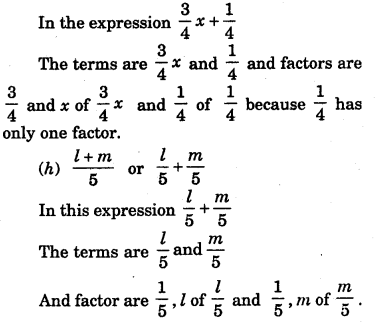(i) 0.1p2 + 0.2q2
In this expression 0.1 p2 + 0.2 q2
The terms are 0.1 p2 and 0.2 q2 and factors are 0.1, p, p of 0.1 p2 and 0.2, q, q of 0.2 q2.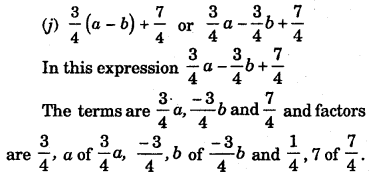Question 3.
Identify the numerical co¬efficients of terms other than constants in the following expressions:
(i) 5-3t2
(ii) 1 + t + t2 + t3
(iii) x + 2xy + 3y
(iv) 100 m + 1000 n
(v) – p2q2 + 7pq
(vi) 1.2a + 0.8 b
(vii) 3.14 r2
(viii) 2(l + b)
(ix) 0.1 y + 0.01 y2.
Solution:

 Expression Terms Numerical Co-efficient (i) 5 – 3t2 -3t2 -3 (ii) 1 + t + t2 + t3 t t2 t3 1 1 1 (iii) x + 2xy + 3y x 2xy 3y 1 2 3 (iv) 100 m + 1000 n 100 m 1000 n 100 1000 (v) – p2q2 + 7pq -(p2q2) 7pq -1 7 (vi) 1.2a + 0.86 1.2a 0.86 1.2 0.8 (vii) 3.14 r2 3.14 r2 3.14 (viii) 2(l + b) = 2l + 2b 2l 2b 2 2 (ix) 0.1y + 0.01 y2 0.1y 0.01y2 0.1 0.01Question 4.
(a) Identify terms which contain x and give the co-efficient of x.
(i) y2x + y
(ii) 13y2 – 8yx
(iii) x + y + 2
(iv) 5 + z + zx
(v) 1 + x +xy
(vi) 12xy2 + 25
(vii) 7x + xy2
(b) Identify terms which contains y2 and give the co-efficient of y2.
(i) 8 – xy2
(ii) 5y2 + 7x
(iii) 2x2y – 15xy2 + 7y2
Solution:
(a)

 Expression Terms – with factor (x) Co-efficient of x (i) y2x + y y2x y2 (ii) 13y2 – 8yx -8yx -8y (iii) x + y + 2 x 1 (iv) 5 + z + zx zx z (v) 1 + x + xy x xy 1 y (vi) 12xy2 + 25 12xy2 12y2 (vii) 7x + xy2 7x xy2 7 y2

(b)

 Expression Terms with factor y2 Co-efficient of y2 (i) 8-xy2 -xy2 – X (ii) 5y2 + 7x 5y2 5 (iii) 2xy2 – 15xy2 + 7y2 2xy2 -15xy2 7y2 2x -15x 7

Question 5.
(i) 4y – 7z
(ii) y2
(iii) x + y – xy
(iv) 100
(v) ab-a-b
(vi) 5 – 3t
(vii) 4p2q – 4pq2
(viii) 7mn
(ix) z2 – 3z + 8
(x) a2 + b2
(xi) z2 + z
(xii) 1 + x + x2
Solution:
(i) 4y – 7z is binomial. It contain 2 terms.
(ii) y2 is monomial. It contains 1 term.
(iii) x + y – xy is trinomial.lt contains 3 terms.
(iv) 100 is monomial. It contains 1 term.
(v) ab-a-b is trinomial.lt contains3 terms
(vi) 5 – 3t is binomial. It contains 2 terms.
(vii) 4p2q – 4pq2 is binomial.lt contains 2 terms.
(viii) 7mn is monomial. It contains 1 term.
(ix) z2 – 3z + 8 is trinomial. It contains 3 terms.
(x) a2 + b2 is binomial. It contain 2 terms.
(xi) z2 + z is binomial. It contains 2 terms.
(xii) 1 + x + x2 is trinomial. It contains 3 terms.Question 6.
State whether the given pair of terms is of like or unlike terms.
(i) 1,100
(ii) -7x, $$\frac{5}{2}$$x
(iii) – 29x, – 29y
(iv) 14xy, 42xy
(v) 4m2p,4mp2
(vi) 12xz, 12x2z2
Solution:

 Pairs Factors Like / Unlike Terms (i) 1, 1 Unlike 100, 100 (ii) – 7x – 7, x 1 5/2 x 5/2, x Like (iii) -29x -29, x -29y – 29, y Unlike (iv) 14 xy 14, x, y 42 yx 42, x, y Like (v) 4m2p 4, m, m, p 4mp2 4, m, p, p Unlike (vi) 12xz 12, x, z 12x2z2 12, x, x, z, z ,       Unlike

Question 7.
Identify like terms in the following:
(a) – xy2, – 4yx2, 8x2, 2xy2, 7y, – 11x2, – 100x, -11yx, 20x2y, -6x2, y, 2xy, 3x.
(b) 10pq, 7p, 8q, – p2q2, – 7qp, – 100q, – 23, 12q2p2, -5P2, 41, 2405p, 78qp, 13p2q, – 9pq2, qp2, 701P2.
Solution: